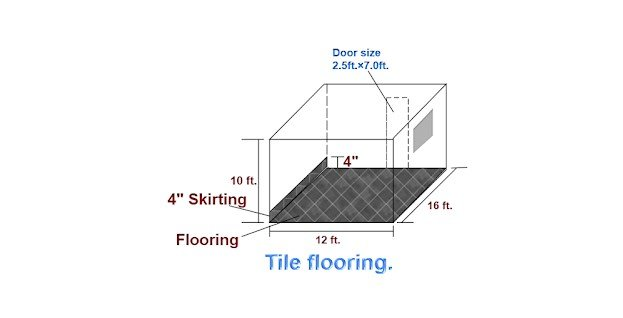Breaking News
Home / Civil Engineering / How To Calculate The Tile Flooring Cost Per Square Feet

# How To Calculate The Tile Flooring Cost Per Square Feet

## How To Calculate The Tile Flooring Cost Per Square Feet

Let us consider a room of size 12 ft. × 16 ft. as shown below.Let us calculate the total cost of fixing the tile for this above-given room.

Given data:

Length of the room = 16ft.

Breadth of the room = 12ft.

Skirting height =  4″ = 0.33ft.

Door size = 2.5 ft × 7 ft.

### Calculation:

1. Area of tile flooring

= 16ft. × 12ft.

192 sqft.

### Area of skirting

= [{(2nos. × length) + (2nos. × breadth) – door width} × height]

= [{(2nos. × 16ft.) + (2nos. × 12ft.) – 2.5 ft.} × 0.33ft.]

= [ { 32ft. + 24ft. – 2.5ft.} × 0.33ft.]

= [ 53.5ft. × 0.33ft.]

17.655 sqft.

### Total area of tile fixing

= area of flooring + area of skirting

= 192 sqft. + 17.66 sqft.

209.66 sqft.

### How to Calculate the Cost of Paint for one Room

Note: Below given data are taken from the above-mentioned article.

Cement bags required for 100sq ft. tile area= 2.84 bag.

The volume of sand required for 100 sq ft. tile area = 0.3953 cum.

By cross multiplication,

1. The number of cement bags required for tile flooring

= [2.84 bag × (209.66 sq ft ÷ 100 sq ft.)]

= [ 2.84 bag × 2.096]

= 5.95 bags.

1. ### The volume of sand required

= [0.3953cum × (209.66 sq ft ÷ 100 sq ft.)]

= [0.3953 cum × 2.096]

= 0.829 cum.

= 29.26 cu ft

1. White cement required for joint filling=1kg. ( Approx.)
1. Labor cost.

The average Labor charge per sq ft of tile work = INR 15/-

The total labor cost

= Total tile area in sq ft. × labor rate/sq ft.

= 209.66 sq ft. × 15/sq ft.

### = 3144.90/-

Now, let us calculate the tile flooring cost in the table format.

 Sl. no. Item. Qty. Rate. in INR. Unit. Cost in INR. 1. Tile 209.66 48/- Sq ft. 10,063.68 2. Cement. 5.95 400/- bags 2,380.00 3. Sand. 0.829 2000/- Cum. 1,658.00 4. White cement. 1.0 20/- Kg. 20.00 5. The material cost = 14,121.68 6. Add 5% wastage = 706.08 7. Total material cost = 14,827.76 8. Labors cost = 3,144.90 9. The total cost of flooring = 17,972.66

Note: All the rates mentioned in the above-given table are the average market rate in India. To get the accurate cost of flooring, replace them with your regional rate.

### Cost per sq ft of tile flooring

=  [Total cost of tile flooring ÷ total tile area]

= [17972.66 ÷ 209.66]

= 85.72/-

By rounding off, the tile flooring cost per sq ft. of the building is INR 86/-

## . Land Surveying & Architects

### THANKS.